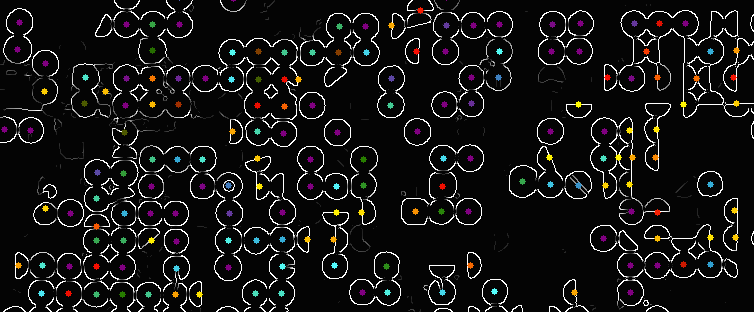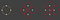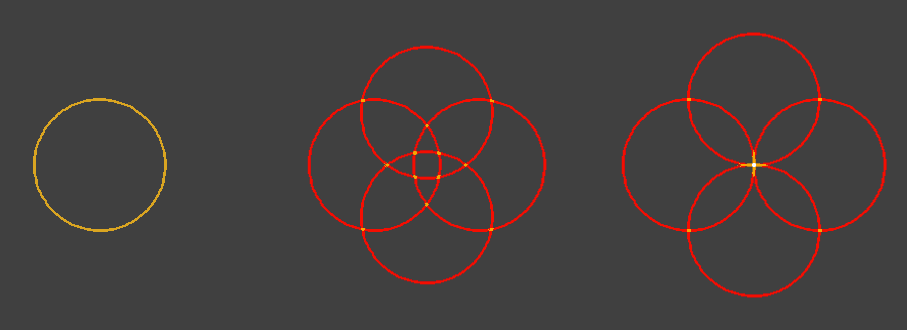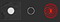# Finding Circles, Revisited

Earth Engine by Example

In the Earth Engine Code Editor Examples, there’s an example of doing circle finding by kernel convolution. This article will demonstrate another method for circle detection that has a little more flexibility called the Circle Hough Transform (CHT).An example of Circle Hough Transform applied to an edge-detected image of center pivot irrigation farms in Southern Colorado. The colored dots represent detected circle centers. Cool colors (purple, aqua, green) indicate better fits, and warmer colors (red, orange, yellow) indicate weaker fits.

The usual recipe for CHT is:

• Smooth the input with a Gaussian convolution
• Perform edge detection with the Canny edge detector
• Iterate over pixels in the image, drawing a circle of a given radius for each input pixel into an accumulator image.
• Find the highest values in the accumulator; these will correspond to the center of any circles in the input.

To see why the CHT works, consider the following diagram where 4 of the pixels in the input circle (left) were traced out as circles with a radius of `0.6*r`(center) and with a radius of `1.0*r` (right).Example of the CHT, tracing out circles and summing for 4 points of the original circle (left) with a radius of 0.6*r (center) and 1.0*r (right).

Where the circles don’t overlap at all, the accumulator has a value of 1. Where they overlap with one other circle, the accumulator has a value of 2, and where they all overlap at the center, there’s a value of 4. That high-point corresponds to the center of the original circle or radius `r`.

In practice, this is done for every angle between 0 and 360 (or some stepped subset) and every possible radius of interest. Then it’s just a matter of finding the maximums in the N-dimensional accumulator space.

The first two steps in the CHT recipe are straightforward in Earth Engine, but it’s impractical to iterate over each pixel in an image there, so we can’t trace and accumulate the circles in the traditional way. Instead, we can approximate this with image displacement, essentially swirling the whole image around in circles of varying sizes and summing them. That looks like this for our example circle and radii of `0.6*r` and `1.0*r`.An approximation of CHT using image displacement instead of tracing. The input image (left) is displaced 4 times and summed with a displacement radius of 0.6*r (center) and 1.0*r (right).

For the “too small” radius of `0.6*r`, the intersection points occur in slightly different location (that we don’t care about), but with the exactly right radius of `1.0*r` we get the same result at the full CHT.

Implementing this is just a matter of mapping over all the radii and angles and summing the intermediate results. Then we can take the max through all of the radii-accumulator images to find the best fit.

Unfortunately, depending on the projection being used and distance from the projection origin, circular objects (or their displacement) might not actually be circles; they can often be ellipses. Below are images showing one center-pivot farm in Southern Colorado (left), its edge-detection result (center), and that edge displaced by the same distance in both X and Y through 12 angles (right). The result is definitely ellipsoidal in this projection due to the location’s northern latitude, and the center “hot spot” has been spread out in the Y direction, making center finding more difficult.An image of a center-pivot field (left), its edges (center), and a visualization of the CHT accumulator (right). The Mercator projection results in elongation of the Y axis at the latitude of this farm.

This is where Hough can outperform other methods: it allows different radii to be used for the X and Y directions independently. The implementation just requires an additional `map()`to accommodate the extra radius.

The whole script, including the Gaussian smoothing, Otsu thresholding binarization, and Canny edge detection, can be found at https://goo.gle/2Rr8Qvw.

# Caveats

• As mentioned, the displacement method is just an approximation of the CHT and at small radii (anything close to 1 pixel), the difference between the two methods is likely to be significant.
• The `displace()` function uses meters at the current location, so the amount of displacement within an image (and the best-fit radii) might actually be more or less pixels, depending on how close a pixel is to the projection’s origin.
• While the binarization threshold is found dynamically, there’s an additional threshold on the results of the Canny edge detection that I arbitrarily set at 0.5.
• In practice, the threshold for what constitutes a good fit depends on the other parameters that have been set (e.g.: number of angles), and determines how complete a circle needs to be to be included. Circles that just touch will have no edge between them, resulting in a lower fit value.